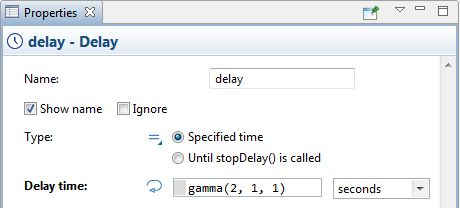AnyLogic

# Choose probability distribution wizard

AnyLogic comes with a large set of probability distributions. To obtain a random value according the probability distribution, you should insert the call of the corresponding function in the expression field, e.g. exponential(1, 0).

AnyLogic provides users with a handy visual wizard that lists and illustrates all the supported probability distributions.

To insert a call of a probability distribution function using the wizard

1. Click in the field where you want to insert a probability distribution function call. If some other function call is already specified there, delete it.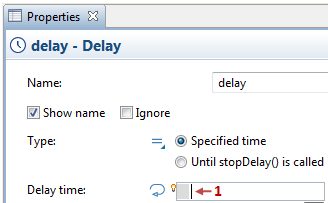2. Click the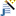Choose Probability Distribution... toolbar button.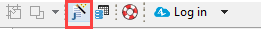3. The Choose Probability Distribution wizard will open: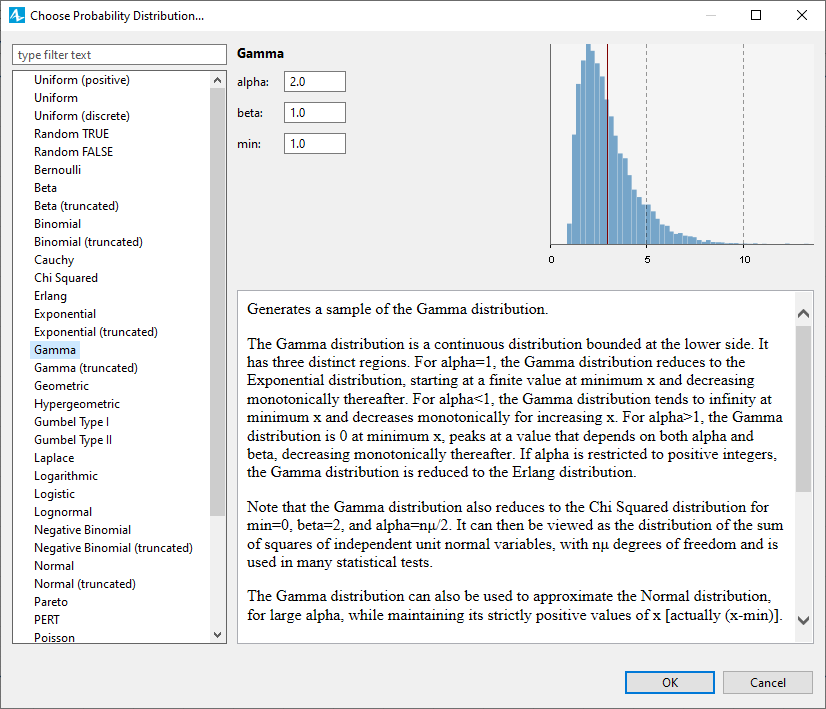4. In the left pane you will see the list of all probability distribution functions supported by AnyLogic. Select the function you need in this list. In the right pane you will see the description, the fields to configure the distribution parameters, and the instantly built preview of the selected distribution.
5. Set the required parameter values, and click OK.
6. You will see the call of the selected probability distribution function inserted in the field: